Covariance

Givensets of variates denoted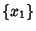, ...,, a quantity called the Covariance Matrix is defined by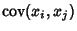(1)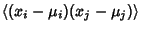(2)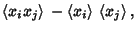(3)

whereandare the Means of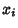and, respectively. An individual element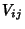of the Covariance Matrix is called the covariance of the two variatesand, and provides a measure of how strongly correlated these variables are. In fact, the derived quantity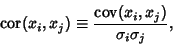(4)

where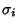,are the Standard Deviations, is called the Correlation ofand. Note that ifandare taken from the same set of variates (say,), then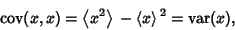(5)

giving the usual Variance. The covariance is also symmetric since(6)

For two variables, the covariance is related to the Variance by(7)

For two independent variates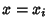and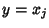,(8)

so the covariance is zero. However, if the variables are correlated in some way, then their covariance will be Nonzero. In fact, if, thentends to increase asincreases. If, thentends to decrease asincreases.

The covariance obeys the identity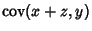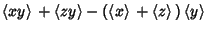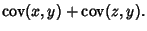(9)

By induction, it therefore follows that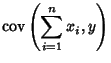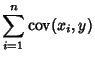(10)(11)(12)(13)(14)